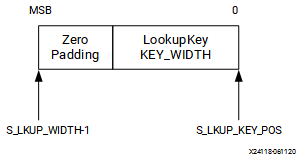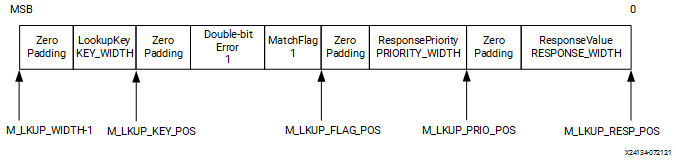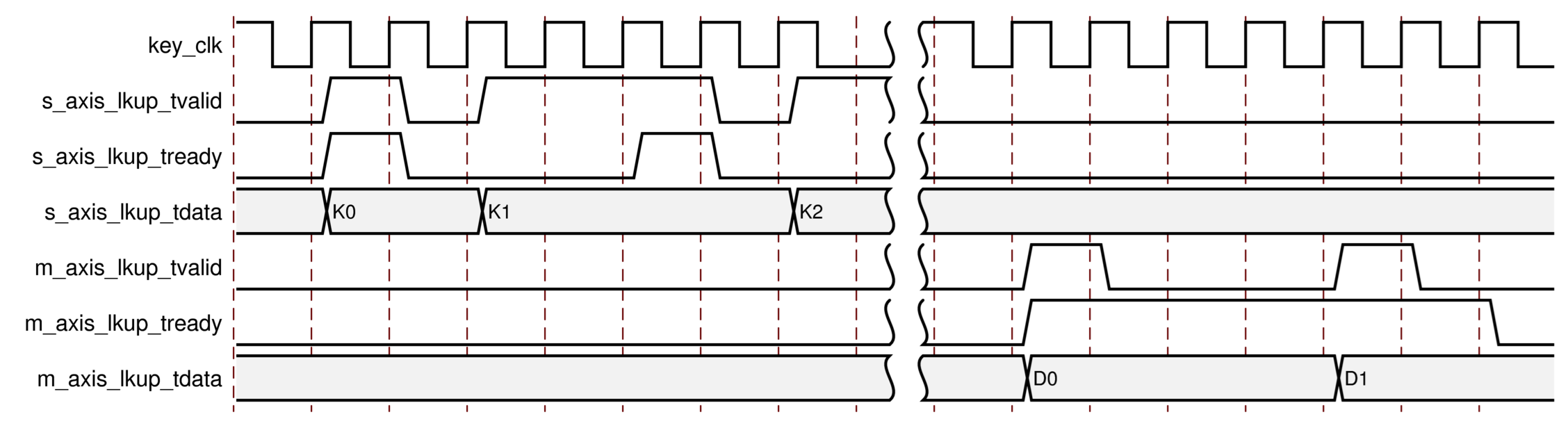# Lookup Interface Ports - 2.3 English

## Ternary CAM Search LogiCORE IP Product Guide (PG318)

Document ID
PG318
Release Date
2022-05-25
Version
2.3 English
Table 1. Lookup Interface
Port Name I/O Clock Description
s_axis_lkup_tdata

[S_LKUP_WIDTH-1:0]

I key_clk The Lookup Request key. tdata is padded with zeros to a byte multiple length.
s_axis_lkup_tready O key_clk tready indicates that the core is ready to accept lookup requests. The ratio of LOOKUP_INTERFACE_FREQ and LOOKUP_RATE determines how frequently new lookups can be started. If LOOKUP_INTERFACE_FREQ equals LOOKUP_RATE than lookups can be started every clock cycle without gaps.
s_axis_lkup_tvalid I key_clk Indicates that the lookup request key is valid. A lookup is initiated when both tready and tvalid are high for one clock cycle.
m_axis_lkup_tdata

[M_LKUP_WIDTH-1:0]

O key_clk The response value associated with the matching entry.

tready for M_AXIS_DATA channel. Tie high if the downstream slave is always able to accept data from M_AXIS_DATA.

m_axis_lkup_tvalid I key_clk tvalid for M_AXIS_DATA channel.
Figure 1. s_axis_lkup_tdataS_LKUP_KEY_POS = 0

S_LKUP_WIDTH = 8 x floor((KEY_WIDTH + 7) / 8)

Figure 2. m_axis_lkup_tdataM_LKUP_RESP_POS = 0

M_LKUP_PRIO_POS = 8 x floor((RESPONSE_WIDTH + 7) / 8)

M_LKUP_FLAG_POS = 8 x (floor((PRIORITY_WIDTH + 7) / 8) + floor((RESPONSE_WIDTH + 7) / 8))[S_LKUP_WIDTH-1:0]

M_LKUP_KEY_POS = 8 x (1 + floor((PRIORITY_WIDTH + 7) / 8) + floor((RESPONSE_WIDTH + 7) / 8))

M_LKUP_WIDTH = 8 x (floor((KEY_WIDTH + 7) / 8) + 2 + floor((PRIORITY_WIDTH + 7) / 8) + floor((RESPONSE_WIDTH + 7) / 8))

Figure 3. Lookup Interface Timing Diagram